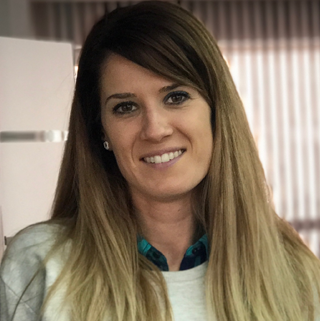Junior

6 - 7 Years
Level 1, Module 1

MindHub - Група 1828

• Date

02/02/2019 (Saturday)

• Durtion

14:30. - 16:30. (2ч) / 4 седмици

• Location

Зала "MindHub - Борово" - ул. Солун 62, ет. 1 Sofia

• Price

120.00 bgn

In this module students are introduced to the world of programming. Students learn what a program is made of and how we can control the behavior of robots and game characters by applying different types of instructions. In this module students learn how ordering instructions in a logical sequence allows us to create programs with clear, exact instructions that lead to an expected result.

Topics в Module 1 (Level 1, Module 1)

First steps into programming
Тема 1

What are an instruction and a program?

In this lesson students are introduced to a basic programming concept – logical sequence. Students learn that computers can execute only precise short instructions that have been ordered in the right way, and that lead to an expected end result. In this lesson students are introduced to the instruction (statement) concept. The practice part of this lesson is based on applying instructions with various tools.

Тема 2

Instructions priority and consecutive actions in creating a program. Using various interfaces

In this lesson students write the sequence of steps that need to be taken for their sprite to move from one place to another. They apply what they have learnt in Lesson 1 in a suitable for them programming environment. Students are briefly introduced to the concept of an event. In this topic students learn what an algorithm is. We teach them that this is a sequence of steps that you take to achieve a pre-defined result. In this lesson students will learn how to use various interfaces to create a set of instructions and will identify the differences between the interfaces.

Тема 3

Basic maths and logical problems

Arithmetic is a key component of the program creation process. In this lesson students find out about the connection between programming and mathematics.

Тема 4

Preparing a project Junior 1/2

This is a practice lesson and it helps students put to use all they have learnt in the module and successfully do a project.

Mentors

•Поли Янкова
: